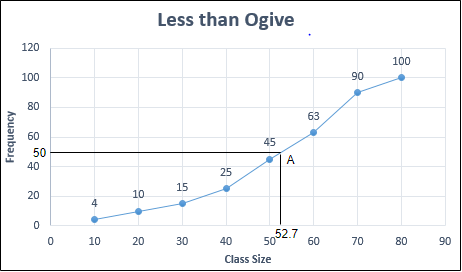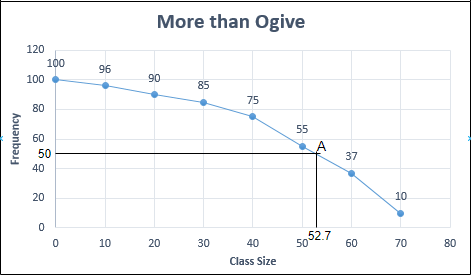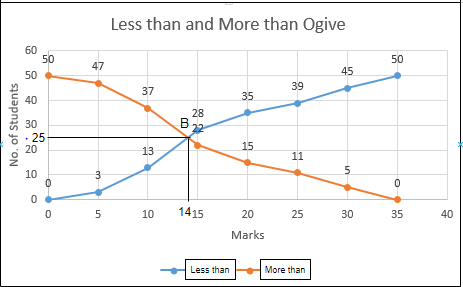# Graphical determination of Median

• Last Updated : 29 Jun, 2022

A measure of central tendency that determines the centrally located value of a given series is known as the Median. The number of values of the series below and above the given series is always equal. To determine the median value of a given series, it is first managed in increasing or decreasing order, then the value in the middle is taken as the central value or median of the series. For example, to determine the Median of the series 2, 20, 14, 18, 4, 11, 15, it will be first written in ascending order, i.e., 2, 4, 11, 14, 15, 18, 20. Now the middle value of this series is the number at 4th place, i.e., 14. Therefore, the median of the given series is 14. The Median is denoted by M.

According to Connor, “The Median is that value of the variable which divides the group into two equal parts, one part comprising all values greater than the median value and the other part comprising all the values smaller than the median value.”

## Graphic Determination of Median

The median of a given series can also be calculated through its graphical representation in the form of less than or more than ogive. Through ogives, the median can be determined in two ways:

1. Presenting the data of given series on a graph in the form of less than ogive or more than ogive.

2. Presenting the data of given series on a graph, simultaneously in the form of both less than ogive and more than ogive. The point where these two ogives meet is the median value of the given series.

### 1. Less than Ogive or More than Ogive Approach

According to the ‘Less than’ or ‘More than’ Ogive Approach, to determine the median of a given series, it is, first of all, converted into either less than or more than cumulative series. After that, the cumulative series are presented graphically for the presentation of less than or more than ogive. In the next step, the N/2th term of the series is determined. From the N/2th term on the graph’s Y-axis, a perpendicular to the right is drawn. This perpendicular cuts the cumulative frequency curve giving the median value of the series.

For example,

Determine median value of the following series with the help of both Less than Ogive Approach and More than Ogive Approach:

Solution:

Using Less Than Ogive Approach

N/2th term = 100/2 = 50

Now, mark 50 on the Y-axis of graph and make a perpendicular from this point to the right to cut the ogive at point A.

From point A, draw a perpendicular on X-axis. The point on X-axis at which the perpendicular cuts, is the median value.The perpendicular from point A cuts the X-axis at point 52.7 .

Therefore, the Median (M) = 52.7

Using More Than Ogive Approach

N/2th term = 100/2 = 50

Now, mark 50 on the Y-axis of graph and make a perpendicular from this point to the right to cut the ogive at point A.

From point A, draw a perpendicular on X-axis. The point on X-axis at which the perpendicular cuts, is the median value.The perpendicular from point A cuts the X-axis at point 52.7 .

Therefore, the Median (M) = 52.7

### 2. Less than Ogive and More than Ogive Approach

According to the ‘Less than’ and ‘More than’ Ogive Approach, to determine the median of a given series, both less than and more than, ogives are presented on a graph simultaneously. The perpendicular on the X-axis drawn from the point at which both the ogive curves cut each other, is the median value of the given series.

For example,

Determine the median value of the following series using the Less than and More than Ogive Approach:

Solution:The less than and more than ogive cuts each other at point B, and the point at which the perpendicular drawn on X-axis from point B is the median value of the given series.

Therefore, the Median (M) = 14

My Personal Notes arrow_drop_up
Recommended Articles
Page :